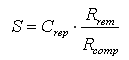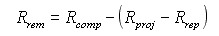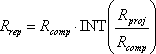# HOMER Pro 3.10

 Navigation: Glossary Salvage ValueSalvage value is the value remaining in a component of the power system at the end of the project lifetime. HOMER assumes linear depreciation of components, meaning that the salvage value of a component is directly proportional to its remaining life. It also assumes that the salvage value depends on the replacement cost rather than the initial capital cost. HOMER calculates salvage value using the following equation:Rrem, the remaining life of the component at the end of the project lifetime, is given by:Rrep, the replacement cost duration, is given by:other definitions: Crep = replacement cost [\$] Rcomp = component lifetime [yr] Rproj = project lifetime [yr] INT() = a function that returns the integer amount of a real number; for example, INT(6.843) = 6

HOMER assumes that salvage value accrues at the end of the project lifetime.

Example 1: A wind turbine has a capital cost of \$1 million, a replacement cost of \$750,000, and a 25-year lifetime. At the end of a 20-year project lifetime, the replacement cost duration, Rrep, is zero, the remaining life, Rrem, is 5 years, so the salvage value is \$750,000 * 5/25 = \$150,000. Note that the capital cost does not affect the calculation of salvage value.

Example 2: A diesel generator has a capital cost of \$400,000, a replacement cost of \$350,000, and a lifetime of 7.85 years. At the end of a 30-year project lifetime, the replacement cost duration, Rrep, is 23.55 years, the remaining life, Rrem, is 1.40 years, so the salvage value is \$350,000 * 1.40/7.85 = \$62,420.

See also

Project Lifetime

Replacement Cost